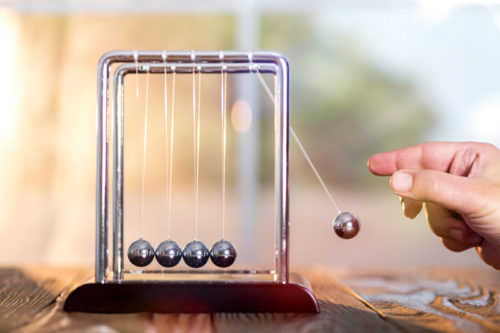# Lower Secondary Physics

## PROGRAMME COVERAGE

### Physics##### Units and Measurement
• Identify the base quantities and their SI units
• Identify and use prefixes and learn how to convert between them
• Learn how to measure a variety of lengths with appropriate accuracy by using metre rules, micrometer screw gauges and vernier calipers, along with how to account for zero errors

##### Forces
• Identify different types of forces and their characteristics
• Calculate weight and pressure to solve related problems

##### Turning Effect of Forces
• Describe and calculate the moment of a force in terms of its turning effect and relate this to everyday examples
• Apply the principle of moments to solve one pivot problems
• Describe different types of levers and their applications

##### Energy
• Define kinetic energy, potential energy (chemical, gravitational, elastic), light energy, thermal energy, electrical energy and nuclear energy state the principle of the conservation of energy and use it to solve related problems
• Understand the concept of conservation of energy and its applications
• Define and calculate work done using their formulas to solve related problems
• Compare the use of non-renewable and renewable energy sources such as fossil fuels, nuclear energy, solar energy, wind energy and hydroelectric generation to generate electricity in terms of energy conversion efficiency, production costs and environmental impact

##### Kinetic Model of Matter
• Understand the macroscopic properties of solids, liquids and gases
• Describe the molecular structure of solids, liquids and gases, relating their properties to the forces and distances between molecules and to the motion of the molecules
• Describe melting/solidification and boiling/condensation
• Explain the difference between boiling and evaporation
##### Heat
• Describe, in molecular terms, how energy transfer occurs in solids
• Describe, in terms of density changes, convection in fluids
• Explain that energy transfer of a body by radiation and how rate of energy transfer is affected by:
(i) colour and texture of the surface
(ii) surface temperature
(iii) surface area
• Apply the concept of thermal energy transfer to everyday applications

##### Waves
• Describe what is meant by wave motion as illustrated by vibrations in ropes and springs and by waves in a ripple tank
• Define speed, frequency, wavelength, period, amplitude and wavefront
• Recall and apply the relevant wave equations
• Define transverse and longitudinal waves and give suitable examples of each

##### Light
• Define and use the terms for reflection, such as normal, angle of incidence and angle of reflection
• Define law of reflection and use this principle in constructions, measurements and calculations
• Define and use the terms for refraction, such as normal, angle of incidence and angle of refraction

##### Sound
• Describe the production of sound and characteristics of sound waves
• Explain how speed of sound differs in gasses, liquids and solids
• Describe a direct method for the determination of the speed of sound in air and make the necessary calculation
• Relate loudness of a sound wave to its amplitude and pitch to its frequency
• Describe how the reflection of sound may produce an echo, and how this may be used for measuring distances
• Define ultrasound and their applications
##### Electricity
• Draw circuit diagrams with power sources, switches, lamps, resistors, fuses, ammeters and voltmeters, bells
• State the relationships of current, electromotive force and potential difference in series and parallel circuits and use them to solve questions
• Describe the use of the heating effect of electricity in appliances such as electric kettles, ovens and heaters
• Use equations of electrical power and energy to solve related problems
• Calculate the cost of using electrical appliances
• State the hazards of using electricity
• Explain the use of fuses and circuit breakers in electrical circuits and calculate fuse ratings
• Explain the need for earthing metal cases and for double insulation
• State the meaning of the terms live, neutral and earth
• Describe the wiring in a mains plug
• Explain why switches, fuses, and circuit breakers are wired into the live conductor